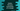# Python program to find the area and perimeter of a triangle## Find the area and perimeter of a triangle in Python :

In this tutorial, I will show you how to find the area and perimeter of a triangle in Python. For calculating both area and perimeter, we need the values of the sides of the triangle. So, the program will take the sides as input from the user and calculate the values.

At the end of this post, I will show you how to use a different class to do the calculations. With this tutorial, you will learn how to read user inputs, how to do basic mathematical calculations, print output on the console, using a class in python etc.

## Perimeter and area of a triangle :

If s1,s2 and s3 are the lengths of three sides of a triangle, its perimeter and area can be calculated using the below two formulae :

``````Perimeter = s1 + s2 + s3
Area = √(s*(s-s1)*(s-s2)*(s-s3))

where s = (s1 + s2 + s3)/2 ``````

## Python program to find the perimeter and area :

``````#1
s1 = float(input("Enter the first side of the triangle : "))
s2 = float(input("Enter the second side of the triangle : "))
s3 = float(input("Enter the third side of the triangle : "))

#2
p = (s1 + s2 + s3)

#3
s = p/2

#4
area = (s * (s-s1) * (s-s2)*(s-s3))**0.5

#5
print("The perimeter of the triangle is : {0:.2f}".format(p))
print("The area of the triangle is : {0:.2f}".format(area))``````

### Explanation :

The commented numbers in the above program denotes the step number below :

1. Get the first, second and third side of the triangle as input from the user. We are reading these values as float instead of integer.
2. Find the perimeter of the triangle.
3. Find the value of s i.e. divide perimeter by 2
4. Find the area. **0.5 is used to find the square root.
5. Finally, print the values of perimeter and area. We are formating the values up to two decimal using 0:.2f.

### Sample output :

``````Enter the first side of the triangle : 9
Enter the second side of the triangle : 10
Enter the third side of the triangle : 11
The perimeter of the triangle is : 30.00
The area of the triangle is : 42.43``````

## Perimeter and area of a triangle using a class :

We can also use one class and one function in it to calculate the perimeter and area :

``````class Util:
def findPerimeter(self, s1, s2, s3):
return (s1 + s2 + s3)

def findArea(self, s1, s2, s3):
p = (s1 + s2 + s3)
s = p/2
return (s * (s-s1) * (s-s2)*(s-s3))**0.5

s1 = float(input("Enter the first side of the triangle : "))
s2 = float(input("Enter the second side of the triangle : "))
s3 = float(input("Enter the third side of the triangle : "))

u = Util()

print("The perimeter of the triangle is : {0:.2f}".format(
u.findPerimeter(s1, s2, s3)))
print("The area of the triangle is : {0:.2f}".format(u.findArea(s1, s2, s3)))``````

It is always a good idea to create one different class to store all utility functions. We can use this class from any other files in the project.

In the above example, Util is a utility class with two functions findPerimeter and findArea. We are creating one new object of this class and calling these methods to find out the perimeter and area with user-provided values.

The output of this program is same as the above example.